# 一种基于历史轨迹数据的实时公交到站预测算法A Real-Time Bus Arrival Time Prediction Algorithm Based on Historical GPS

• 全文下载: PDF(747KB)    PP.222-228   DOI: 10.12677/OJTT.2018.74028
• 下载量: 327  浏览量: 1,035   科研立项经费支持

Real-time bus arrival time prediction is an important part of smart transit service. Reliable pre-dicting results can help passengers to reduce waiting time and plan trip reasonably. Common al-gorithms have many problems, such as many kinds of data needed, complex models, single application scenarios and high complexity of algorithms, this paper presents a real-time bus arrival time prediction algorithm based on historical GPS, providing passengers with remaining travel time, number of stations and distance. And an Android application accessing real-time GPS data in Shenzhen City is developed to verify the accuracy and simplicity of the proposed algorithm to meet the needs of large-scale application.

1. 研究背景

2. 算法思路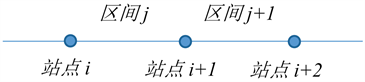Figure 1. Line interruption

2.1. 历史数据处理

1) 数据清洗：对历史轨迹数据中位置异常和重复、无效记录进行清洗，将部分有信息缺失的记录根据其他记录进行补齐；

2) 轨迹匹配：根据轨迹点位置和方向角信息判断车辆运行方向，并匹配至公交线路最邻近点上(图2)；

3) 到站时间插值：搜索站点邻近轨迹点，对历史到站时间进行插值。为了简化估计过程，本文假设相邻轨迹点间车辆匀速运行，从而根据距离插值得到站点的到达时间。如图3所示，A、B为相邻两个轨迹点，记录时间分别为 ${t}_{A}$${t}_{B}$ ，中间经过站点x，则到达x的时间

${t}_{x}={t}_{A}+\left({t}_{B}-{t}_{A}\right)\ast {d}_{Ax}/{d}_{AB}$

4) 路段行程时间：计算物理区间内行程时间，并根据工作日、非工作日，以1个小时为粒度求得各时间段的平均行程时间 ${t}_{AB}^{i}$ ，i为第i个时间段(图4)。

2.2. 实时预测

${t}_{{P}^{\prime },i+1}={t}_{i,i+1}\ast {d}_{{P}^{\prime },i+1}/{d}_{i,i+1}$

${T}_{n}={t}_{{P}^{\prime },i+1}+{t}_{i,i+1}+\cdots +{t}_{n-1,n}$

3. 算法应用(a) 匹配前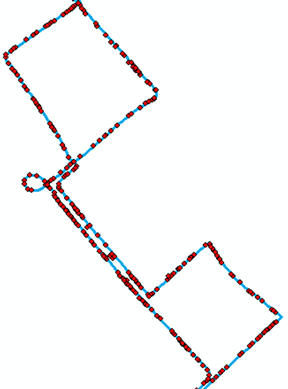(b) 匹配后

Figure 2. Schematic diagram of trace matchingFigure 3. Arrival time interpolation diagram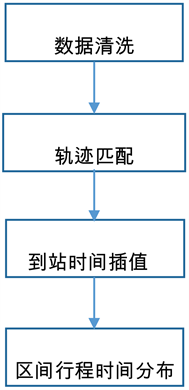Figure 4. Historical data processing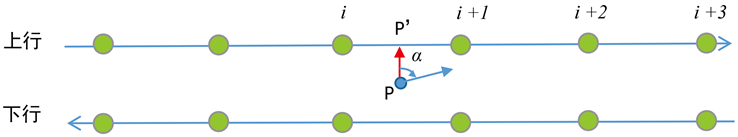Figure 5. Real-time location acquisition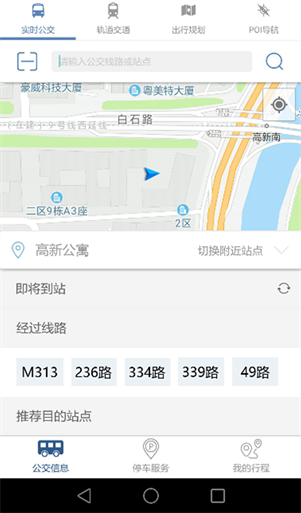Figure 6. User interface

3.1. 数据表设计

3.2. 算法效率分析

3.3. 实测验证

4. 总结与展望

1) 对于行程时间预测的精细度有待进一步提高，加入车辆停靠时间、信号灯导致的延迟等因素  ；

2) 对于异常突发事件如事故、台风天气导致的车辆延迟情况，需要在算法中加入异常处理模块。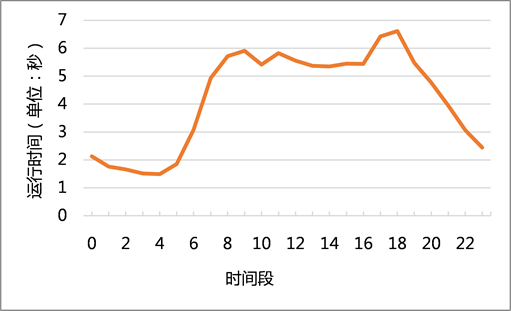Figure 7. Algorithm efficiency analysis

  沈旗. 基于浮动公交车的交通信息实时检测和公交车到站时间预测研究[D]: [硕士学位论文]. 杭州: 浙江大学, 2007.  向红艳, 彭学文. 公交到站时间预测研究现状与发展趋势[J]. 交通信息与安全, 2014, 32(4): 57-61.  王锐, 杨宏业, 张海鹏. 基于浮动公交车的SVM实时交通状态识别研究[J]. 内蒙古工业大学学报, 2016, 35(1): 18-23.  胡华, 高云峰, 刘志钢. 基于AVL数据的公交到站时间实时预测模型[J]. 重庆交通大学学报(自然科学版), 2012, 31(5): 1014-1017.  孙棣华, 赖云波, 廖孝勇, 等. 公交浮动车辆到站时间实时预测模型[J]. 交通运输工程学报, 2011(2): 84-89.